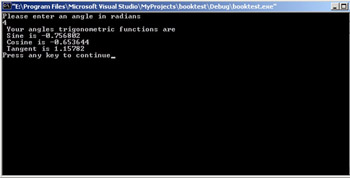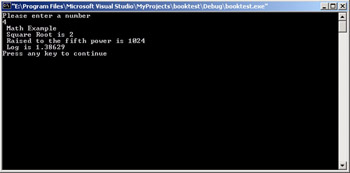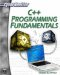# Math Operations

C++ has a rich set of mathematical operations that are at your disposal. Learning to use these will be quite useful to you as we move throughout this book. To utilize these functions you need to include the math header file.

`#include <cmath>`

This header file includes a lot of math functions that are very useful. The most commonly used functions are listed in Table 4.2.

Table 4.2: Math Functions

Function

Purpose

double cos(double);

This function takes an angle (as a double) and returns the cosine.

double sin(double);

This function takes an angle (as a double) and returns the sine.

double tan(double);

This function takes an angle (as a double) and returns the tangent.

double log(double);

This function takes a number and returns the natural log of that number.

double pow(double, double);

With this function, you pass it two numbers. The first is a number you wish to raise and the second is the power you wish to raise it to.

double hypot(double, double);

If you pass this function the length of two sides of a right triangle, it will return you the length of the hypotenuse.

double sqrt(double);

You pass this function a number and it gives you this square root.

int abs(int);

This function returns the absolute value of an integer that is passed to it.

double fabs(double)

This function returns the absolute value of any decimal number passed to it.

double floor(double)

Finds the integer which is less than or equal to the argument passed to it.

 Hint! In this table you probably noticed that the function arguments are listed only as types, with no name. When you call the functions you will pass it the name of whatever variable you wish to pass it. What that function internally calls the argument is irrelevant. What is important is remembering what type to pass it and what type it will return.

There are many other math functions available to you when you include this header file, but the functions shown in Table 4.2 are the most commonly used. The following example illustrates some of these functions.

### Example 4.8

Step 1: Enter the following code into your favorite text editor.

`#include <iostream> #include <cmath>//Math.h is the old C style math   //header file using namespace std; int main() { double angle, dsine,dcos,dtan; cout << "Please enter an angle in radians \n"; cin >> angle; dcos =cos(angle); dsine =sin(angle); dtan =tan(angle); cout << " Your angles trigonometric functions are  \n"; cout << " Sine is " << dsine << "\n"; cout << " Cosine is " << dcos << "\n"; cout << " Tangent is " << dtan << "\n"; return 0;    }// main`

Step 2: Compile the code.

Step 3: When you run the code you should see something like what is shown in Figure 4.8.Figure 4.8: Math functions.

You can see that trigonometry is quite easy with C++ math functions. Although not covered here, that math.h header file also has hyperbolic trigonometric functions and arc functions.

In addition to trigonometry functions, you have a number of standard math functions at your disposal. You should have noticed some of these listed in Table 4.2. The following example illustrates some of these functions.

### Example 4.9

Step 1: Enter the following code into your favorite text editor.

`#include <iostream> #include <cmath> using namespace std; int main() {  double number, dsqrt,dpow,dlog;  cout << "Please enter a number \n";  cin >> number;  dsqrt =sqrt(number);  dpow =pow(number,5);  dlog =log(number);  cout << " Math Example\n";  cout << " Square Root is " << dsqrt << "\n";  cout << " Raised to the fifth power is " << dpow <<    "\n";  cout << " Log is " << dlog << "\n";  return 0;    }// main`

Step 2: Compile the code.

Step 3: Run the code. You should see something similar to the image in Figure 4.9.Figure 4.9: More math functions.

As you can see, most mathematics is quite simple using C++. It is not uncommon to find that mathematicians who take up programming as a hobby often choose C++ as their language of choice. Chapter 3 demonstrated how easily C++ handles binary numbers, and now you have seen that it has a number of math functions already defined.C++ Programming Fundamentals (Cyberrookies)
ISBN: 1584502371
EAN: 2147483647
Year: 2005
Pages: 197
Authors: Chuck Easttom